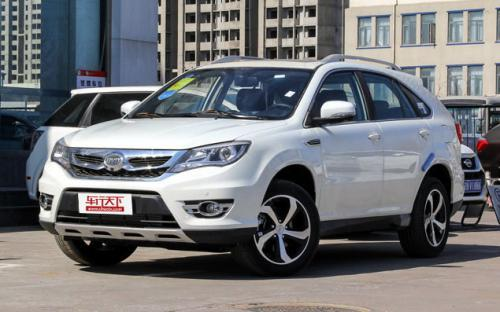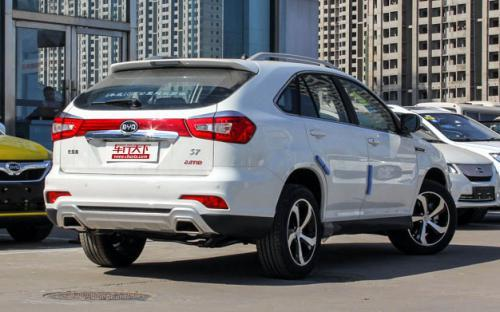### 比亚迪 比亚迪S76 种颜色可选2016款最低售价：10.69 万元起

4835(mm)1855(mm)1720(mm)##### 配置亮点：
• 胎压监测装置

• ISOFIX儿童座椅接口

• 车身稳定控制(ESC/ESP/DSC等)

• 电动天窗

• 定速巡航

• 后倒车雷达

• 真皮座椅

• GPS导航系统

• 氙气大灯

• 后视镜加热

• 提交
2016款 2.0T 自动尊贵型 (134张)
• 2016款 2.0T 自动尊贵型 (134张)
• 2016款 2.0T 自动旗舰型 (109张)
• 2016款 2.0T 自动旗舰型Plus (145张)
• 2016款 1.5T 手动尊贵型 (134张)
• 比亚迪 比亚迪S7 绕车实拍• 比亚迪 比亚迪S7 在售车型

排量 车型 厂商指导价 本地最低报价 购车工具
1.5T
10.69万
10.69万

11.69万
2.0T 自动豪华型 6挡双离合
11.99万
11.99万

2.0T 自动尊贵型 6挡双离合
12.99万
2.0T 自动旗舰型 6挡双离合
13.99万
2.0T 自动尊贵型Plus 6挡双离合
13.69万
2.0T 自动旗舰型Plus 6挡双离合
14.69万
14.69万

2.0T
10.69万
10.69万

11.69万
2.0T 自动豪华型 6挡双离合
11.99万
11.99万

2.0T 自动尊贵型 6挡双离合
12.99万
2.0T 自动旗舰型 6挡双离合
13.99万
2.0T 自动尊贵型Plus 6挡双离合
13.69万
2.0T 自动旗舰型Plus 6挡双离合
14.69万
14.69万

比亚迪 比亚迪S7 经销商

查看更多 >>

### 比亚迪 比亚迪S7 动力加速

比亚迪S7 0-100公里加速时间分布在 0.0-秒 属于 超跑级

动力级别 加速时间 车型

比亚迪 比亚迪S7 视频

比亚迪 比亚迪S7 新闻资讯

# 高颜值长城WEY VV7油耗高？与比亚迪冠道三车远途拼油耗

试驾评测 超过13890次关注

8月27日播出的第七评测评车辆为——长城旗下豪华品牌WEYVV72.0T两驱旗舰版。这款在众多网友眼中外观霸气、颜值不错的“魏派VV7”，自上市以来却被吐槽“油耗作假”...

# 14万买七座SUV 比亚迪S7对比吉利豪情SUV

试驾评测 超过9140次关注

二胎到来，普通的SUV已经满足不了这类家庭了，今天我们推荐两款七座中型SUV，最重要的是他们的价格还非常实惠。说到这儿，各位肯定等不及要知道了。我要推荐给大家...

# 售价13.69-14.69万 比亚迪S7新车型上市

新闻 超过9092次关注

日前，比亚迪汽车正式宣布，旗下中型SUV比亚迪S7推出2.0TID7座尊贵型Plus和2.0TID7座旗舰型Plus两款新车型，新车的售价区间为13.69-14.69万元。

# 比亚迪S7将增1.5T车型 明年上半年上市

国产新车 超过4648次关注

日前，据国内媒体报道，比亚迪S7将会推出1.5L涡轮增压动力车型，传动系统匹配手动变速箱，有望2015年上半年上市。

# 比亚迪S7将10月20日上市 预售15万左右

国产新车 超过6413次关注

近日获悉，比亚迪将会在10月20日公布S7的市场指导价格，同时宣布上市。据悉，新车的预售价格为15万元左右。

猜你喜欢

﻿
• 快速找车
• 选择品牌
• 选择品牌
• A  奥迪
• A  阿斯顿·马丁
• A  阿尔法·罗密欧
• B  宝沃
• B  布加迪
• B  巴博斯
• B  保时捷
• B  宾利
• B  奔驰
• B  宝马
• B  本田
• B  别克
• B  标致
• B  比亚迪
• B  宝骏
• B  北汽制造
• B  北汽新能源
• B  北汽幻速
• B  北汽威旺
• B  北京汽车
• B  奔腾
• B  北汽绅宝
• C  长安
• C  长安商用
• C  长城
• C  昌河
• D  大众
• D  道奇
• D  DS
• D  东南
• D  东风风神
• D  东风风行
• D  东风小康
• D  东风风度
• D  东风
• F  福特
• F  丰田
• F  菲亚特
• F  法拉利
• F  福田
• F  福迪
• F  福汽启腾
• G  观致
• G  广汽传祺
• G  广汽吉奥
• G  GMC
• H  红旗
• H  汉腾汽车
• H  哈弗
• H  哈飞
• H  海格
• H  海马
• H  华颂
• H  黄海
• H  华泰
• H  恒天
• J  吉利汽车
• J  捷豹
• J  Jeep
• J  江淮
• J  江铃
• J  金杯
• J  九龙
• J  金旅
• K  凯翼
• K  凯迪拉克
• K  克莱斯勒
• K  科尼塞克
• K  卡威
• K  开瑞
• L  路虎
• L  林肯
• L  劳斯莱斯
• L  兰博基尼
• L  雷克萨斯
• L  铃木
• L  雷诺
• L  理念
• L  力帆
• L  莲花汽车
• L  猎豹
• L  路特斯
• L  陆风
• M  马自达
• M  MG
• M  MINI
• M  玛莎拉蒂
• M  摩根
• M  迈凯轮
• N  纳智捷
• O  欧宝
• O  讴歌
• O  欧朗
• Q  奇瑞
• Q  起亚
• Q  启辰
• R  日产
• R  荣威
• R  瑞麒
• S  三菱
• S  斯威汽车
• S  萨博
• S  smart
• S  斯柯达
• S  斯巴鲁
• S  思铭
• S  双龙
• S  上汽大通
• S  双环
• T  特斯拉
• T  腾势
• W  沃尔沃
• W  五菱汽车
• W  五十铃
• W  威兹曼
• W  威麟
• X  现代
• X  雪佛兰
• X  雪铁龙
• X  西雅特
• Y  一汽
• Y  英菲尼迪
• Y  英致
• Y  依维柯
• Y  野马汽车
• Y  永源
• Z  众泰
• Z  中华
• Z  中兴
• Z  知豆
• 选择车系
• 选择车系
• 车型对比
• 选择品牌
• 选择品牌
• A  奥迪
• A  阿斯顿·马丁
• A  阿尔法·罗密欧
• B  宝沃
• B  布加迪
• B  巴博斯
• B  保时捷
• B  宾利
• B  奔驰
• B  宝马
• B  本田
• B  别克
• B  标致
• B  比亚迪
• B  宝骏
• B  北汽制造
• B  北汽新能源
• B  北汽幻速
• B  北汽威旺
• B  北京汽车
• B  奔腾
• B  北汽绅宝
• C  长安
• C  长安商用
• C  长城
• C  昌河
• D  大众
• D  道奇
• D  DS
• D  东南
• D  东风风神
• D  东风风行
• D  东风小康
• D  东风风度
• D  东风
• F  福特
• F  丰田
• F  菲亚特
• F  法拉利
• F  福田
• F  福迪
• F  福汽启腾
• G  观致
• G  广汽传祺
• G  广汽吉奥
• G  GMC
• H  红旗
• H  汉腾汽车
• H  哈弗
• H  哈飞
• H  海格
• H  海马
• H  华颂
• H  黄海
• H  华泰
• H  恒天
• J  吉利汽车
• J  捷豹
• J  Jeep
• J  江淮
• J  江铃
• J  金杯
• J  九龙
• J  金旅
• K  凯翼
• K  凯迪拉克
• K  克莱斯勒
• K  科尼塞克
• K  卡威
• K  开瑞
• L  路虎
• L  林肯
• L  劳斯莱斯
• L  兰博基尼
• L  雷克萨斯
• L  铃木
• L  雷诺
• L  理念
• L  力帆
• L  莲花汽车
• L  猎豹
• L  路特斯
• L  陆风
• M  马自达
• M  MG
• M  MINI
• M  玛莎拉蒂
• M  摩根
• M  迈凯轮
• N  纳智捷
• O  欧宝
• O  讴歌
• O  欧朗
• Q  奇瑞
• Q  起亚
• Q  启辰
• R  日产
• R  荣威
• R  瑞麒
• S  三菱
• S  斯威汽车
• S  萨博
• S  smart
• S  斯柯达
• S  斯巴鲁
• S  思铭
• S  双龙
• S  上汽大通
• S  双环
• T  特斯拉
• T  腾势
• W  沃尔沃
• W  五菱汽车
• W  五十铃
• W  威兹曼
• W  威麟
• X  现代
• X  雪佛兰
• X  雪铁龙
• X  西雅特
• Y  一汽
• Y  英菲尼迪
• Y  英致
• Y  依维柯
• Y  野马汽车
• Y  永源
• Z  众泰
• Z  中华
• Z  中兴
• Z  知豆
• 选择车系
• 选择车系
• 选择车型
• 选择车型
• 意见反馈